• Otsu方法有一个重要的特性，就是它完全以在一幅图像的直方图上执行计算为基础，而直方图是很容易得到的一维阵列。  具体的公式推理及公式细节就不说了，详见 Conzalez 那本书，我是第三版的，在P.479——P.482 ...
    Otsu法是1979年由日本大津提出的。该方法在类间方差最大的情况下是最佳的，即统计鉴别分析中所用的度量。Otsu方法有一个重要的特性，就是它完全以在一幅图像的直方图上执行计算为基础，而直方图是很容易得到的一维阵列。
具体的公式推理及公式细节就不说了，详见 Conzalez 那本书，我是第三版的，在P.479——P.482 上面。
给出具体步骤如下：
1、计算输入图像的直方图，并归一化。
2、计算累积均值mu，以及全局灰度均值。
3、计算被分到类1的概率q1，和被分到类2的概率q2。
4、用公式计算类间方差，sigma = q1*q2*(mu1 - mu2)*(mu1 - mu2)
5、循环寻找类间方差最大值，并记下此时的阈值，即为最佳阈值。
6、利用最佳阈值进行图像阈值化。
关于otsu部分的程序代码如下：
double getThreshVal_Otsu_8u( const Mat& _src )
{
Size size = _src.size();
const int N = 256;
int i, j, h[N] = {0};
unsigned char* src;
//直方图统计
for( i = 0; i < size.height; i++ )
{
src = _src.data + _src.step*i;
j = 0;
for(j = 0; j < size.width; j++ )
h[src[j]]++;
}
//像素平均值
double mu = 0, scale = 1./(size.width*size.height);
for( i = 0; i < N; i++ )
{
mu += i*(double)h[i];//累加均值
}
mu *= scale;//平均

double mu1 = 0, q1 = 0;//q1 ,q2 为类1和类2的概率累积和，mu1=mg*q1
double p_i, q2, mu2, sigma;
double max_sigma = 0, max_val = 0;
//循环求取最大阈值
for( i = 0; i < N; i++ )
{
p_i = h[i]*scale;//直方图归一化
mu1 *= q1;
q1 += p_i;
q2 = 1. - q1;
mu1 = (mu1 + i*p_i)/q1;
mu2 = (mu - q1*mu1)/q2;
sigma = q1*q2*(mu1 - mu2)*(mu1 - mu2);//类间方差
if( sigma > max_sigma )
{
max_sigma = sigma;
max_val = i;//记下使类间方差最大的阈值
}
}
return max_val;//返回阈值
}
注意，上面这部分传递的图像是 Mat 类型的，和 Iplimage 类型在图像参数部分写法上有一点区别，注意区分开来
显示结果如下：展开全文图像处理 计算机视觉 算法 阈值分割
• OTSU算法是由日本学者OTSU于1979年提出的一种对图像进行二值化的高效算法。（大津算法） Otsu原理 对于图像 t (x,y)，前景(即目标)和背景的分割阈值记作 T，属于前景的像素点数占整幅图像的比例记为 ω0，平均灰度为...
概念
OTSU算法是由日本学者OTSU于1979年提出的一种对图像进行二值化的高效算法。（大津算法）
Otsu原理
对于图像 t (x,y)，前景(即目标)和背景的分割阈值记作 T，属于前景的像素点数占整幅图像的比例记为 ω0，平均灰度为 μ0；背景像素点数占整幅图像的比例为 ω1，平均灰度为 μ1；整幅图像的平均灰度记为μ，类间方差记为g。
假设图像大小为M×N，图像中像素的灰度值小于阈值 T 的像素个数为 N0，像素灰度大于阈值T的像素个数为 N1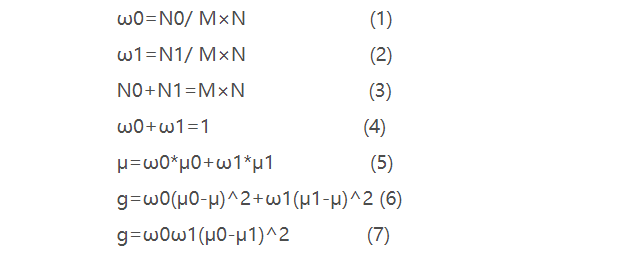注：（7）式是将（5）式代入（6）式得到的，我们的重点放在（7）式上。
Otsu用处
利用Otsu算法，我们可以得到一个阈值，利用该阈值对图像进行二值化等操作。相比于单阈值的固定阈值，otsu算法效果更好。
MATLAB中实现Otsu算法的是 garythresh()函数，一般都与im2bw()配套使用
例：
t=rgb2gray(imread('a1.jpg'));
x=graythresh(t);%获取otsu算得的阈值
t=im2bw(t,x);

graythresh()源码–MATLAB
function [level em] = graythresh(I)
%GRAYTHRESH Global image threshold using Otsu's method.
%   LEVEL = GRAYTHRESH(I) computes a global threshold (LEVEL) that can be
%   used to convert an intensity image to a binary image with IM2BW. LEVEL
%   is a normalized intensity value that lies in the range [0, 1].
%   GRAYTHRESH uses Otsu's method, which chooses the threshold to minimize
%   the intraclass variance of the thresholded black and white pixels.
%
%   [LEVEL EM] = GRAYTHRESH(I) returns effectiveness metric, EM, as the
%   second output argument. It indicates the effectiveness of thresholding
%   of the input image and it is in the range [0, 1]. The lower bound is
%   attainable only by images having a single gray level, and the upper
%   bound is attainable only by two-valued images.
%
%   Class Support
%   -------------
%   The input image I can be uint8, uint16, int16, single, or double, and it
%   must be nonsparse.  LEVEL and EM are double scalars.
%
%   Example
%   -------
%       level = graythresh(I);
%       BW = im2bw(I,level);
%       figure, imshow(BW)
%
narginchk(1,1);
validateattributes(I,{'uint8','uint16','double','single','int16'},{'nonsparse'}, ...
mfilename,'I',1);

if ~isempty(I)
% Convert all N-D arrays into a single column.  Convert to uint8 for
% fastest histogram computation.
I = im2uint8(I(:));
num_bins = 256;
counts = imhist(I,num_bins);

% Variables names are chosen to be similar to the formulas in
% the Otsu paper.
p = counts / sum(counts);
omega = cumsum(p);
mu = cumsum(p .* (1:num_bins)');
mu_t = mu(end);

sigma_b_squared = (mu_t * omega - mu).^2 ./ (omega .* (1 - omega));

% Find the location of the maximum value of sigma_b_squared.
% The maximum may extend over several bins, so average together the
% locations.  If maxval is NaN, meaning that sigma_b_squared is all NaN,
% then return 0.
maxval = max(sigma_b_squared);
isfinite_maxval = isfinite(maxval);
if isfinite_maxval
idx = mean(find(sigma_b_squared == maxval));
% Normalize the threshold to the range [0, 1].
level = (idx - 1) / (num_bins - 1);
else
level = 0.0;
end
else
level = 0.0;
isfinite_maxval = false;
end

% compute the effectiveness metric
if nargout > 1
if isfinite_maxval
em = maxval/(sum(p.*((1:num_bins).^2)') - mu_t^2);
else
em = 0;
end
end

Ostu算法（个人实现 MATLAB版）
t=rgb2gray(imread('a1.jpg'));
[m,n]=size(t);

%counts为图片总像素个数值
counts=m*n;

%count是一个256行一列的矩阵 记录了每个灰度级上像素点的个数
count=imhist(t);

%每个灰度级上像素点占总像素点数量的比例（概率）
p=count/counts;

%cumsum 计算累加概率
w0=cumsum(p);

%u  计算的是平均灰度  比如灰度级为0~125的平均灰度值
%(1:256)'  矩阵转置 1行256列转换为256行1列
u=cumsum(p.*(1:256)');

%u_end是全局平均灰度
u_end=u(end);

%d 求方差 d是256行一列的矩阵
d=(w0*u_end-u).^2./(w0.*(1-w0));

%在d中寻找为最大方差的灰度值 这里y是最大方差的灰度值
[x,y]=max(d);

%为了和im2bw配合使用 进行归一化
%x就是所得阈值
x=(y-1)/255

算法结果验证
t=rgb2gray(imread('a1.jpg'));
[m,n]=size(t);
counts=m*n;
count=imhist(t);
p=count/counts;
w1=cumsum(p);
u=cumsum(p.*(1:256)');
u_end=u(end);%u_end是全局平均灰度
d=(w1*u_end-u).^2./(w1.*(1-w1));
[x,y]=max(d);
x=(y-1)/255 %x就是所得阈值
xx=graythresh(t)%graythresh计算出的阈值

结果
%自己编写的代码计算出的阈值
x =

0.7569

%利用garythresh计算出的阈值
xx =

0.7569

结论
该代码与gragthresh（）计算出的阈值大体上完全一致！
疑点解惑
在我们自己编写的代码中，计算方差用的是下面的公式，
与原理中计算方差的公式（ g=ω0ω1(μ0-μ1)^ ）不一样
难道是原理错了？
哈哈
不是，其实都是一个公式，我们在代码中的公式只是原理公式的变形罢了，这是为了减少变量，提高运算速度。
%d 求方差 d是256行一列的矩阵
d=(w1*u_end-u).^2./(w1.*(1-w1));

公式变形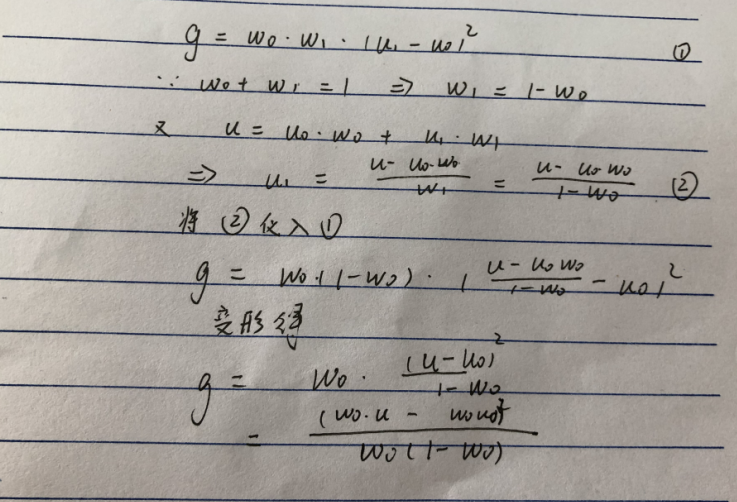注意：上图中的u就是验证代码中的u_end,代表的是图像全局的平均灰度
而u是代表灰度值	T前面的平均灰度。
这里要注意图中的w0u0就是验证代码中的u，对每个灰度级数乘以对应的概率并累加，这是因为u0的计算公式是每个灰度级乘以对应的数量，再除以前面一共的像素数量，这里前面一共的像素数量可以用总量乘以前面占的概率w0。哈哈，对，就是这里，就可以把w0u0中的w0约掉，所以w0*u0==u（这里的u是验证代码中的u，代表灰度值乘以概率的累加值）
其实只需要知道g=w0w1(u1-u0)^2就行了，验证代码只是为了减少变量，做了一下变形，哎，数学还是要学好啊
下面在给个版本的验证算法，原理差不多，只是个别地方做了精简（其实现在看来，这个算法反而搞复杂了）
C=imread('h.jpg');
C=rgb2gray(C);
%读取图像

figure,imshow(C);title('原始灰度图像');%绘原图

count=imhist(C);                       %直方图统计
subplot(1,3,1);
imhist(C);                  %绘制直方图

[r,t]=size(C);

%图像矩阵大小

N=r*t;                                 %图像像素个数

L=256;                                 %制定凸显灰度级为256
count=count/N;
%各级灰度出现概率

for i=2:L
if count(i)~=0  ;
st=i-1;
break
end
end
%以上循环语句实现寻找出现概率不为0的最小灰度值
for i=L:-1:1

if count(i)~=0;

nd=i-1;

break

end

end

%实现找出出现概率不为0的最大灰度值
f=count(st+1:nd+1);

p=st;q=nd-st;
%p和q分别是灰度的起始和结束值

u=0;

for i=1:q

u=u+f(i)*(p+i-1);

ua(i)=u;

end
%计算图像的平均灰度值
for i=1:q

w(i)=sum(f(1:i));

end

%计算出选择不同k的时候，A区域的概率

d=(u*w-ua).^2./(w.*(1-w));   %求出不同k值时类间方差

[y,tp]=max(d);                %求出最大方差对应的灰度值

th=tp+p;
if th<=160
th=tp+p;
else
th=160
end                            %根据具体情况适当修正门限

Y1=zeros(r,t);
for i=1:r

for j=1:t
X1(i,j)=double(C(i,j));

end
end
for i=1:r
for j=1:t
if (X1(i,j)>=th)
Y1(i,j)=X1(i,j);
else Y1(i,j)=0;
end
end
end
%上面一段代码实现分割
figure,imshow(Y1);title('灰度门限分割图像');



展开全文MATLAB 数字图像处理 otsu算法 最大类间方差法
• 图像处理实践中，将灰度图转化为二值图是非常常见的一种预处理手段。在Matlab中，可以使用函数BW = im2bw(I, level)来将一幅灰度图 I，转化为二值图。其中，参数level是一个介于0~1之间的值，也就是用于分割图像的...
在图像处理实践中，将灰度图转化为二值图是非常常见的一种预处理手段。在Matlab中，可以使用函数BW = im2bw(I, level)来将一幅灰度图 I，转化为二值图。其中，参数level是一个介于0~1之间的值，也就是用于分割图像的阈值。默认情况下，它可取值是0.5。

现在问题来了，有没有一种根据图像自身特点来自适应地选择阈值的方法呢？答案是肯定的！我们今天就来介绍其中最为经典的Otsu算法（或称大津算法）。该算法由日本科学家大津展之（Nobuyuki Otsu）于1979年提出。这个算法看似简单，却与统计分析中的“方差分析”方法有很深的渊源。有兴趣的读者也可以参考算法原文《A threshold selection method from gray-level histograms》（在线浏览地址：http://wenku.baidu.com/view/996e972d7375a417866f8f5d）我们今天在介绍该算法原理的基础之上，通过简单的Matlab代码来演示它的实现。

Matlab的帮助信息中指出：To compute the level argument,you can use the function graythresh. The graythresh function uses Otsu's method。可见，函数graythresh()就是Matlab中的大津法实现。如果对Otsu算法的原理并不感兴趣，完全可以直接调用graythresh()函数，而无需过多关系其中的技术细节。

在大津法中，我们定义组内方差为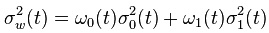通过选择使得上述组内方差最小化时的阈值 t，就可以使得图像中的前景和背景尽可能的被区别开（假设我们将最终图像里被分开的两部分称为前景和背景）。w0和w1分别是一个像素可能属于前景或背景的概率，而 σ 表示两个类别的方差。如果一个图像的直方图有L个等级（一般L=256），那么在给定阈值 t的情况下，w0和w1分别定义为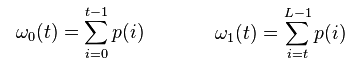大津展之证明最小化组内方差（intra-class variance）与最大化组间方差（inter-class variance）是等价的，于是有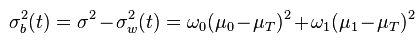又因为（其中 μ 表示均值或期望）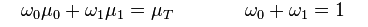可以推出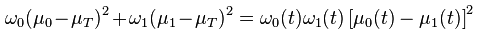这个证明仅仅涉及一些算术上的推导，我简单演示如下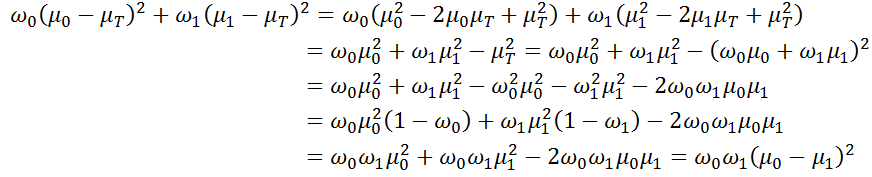最后我们给出在Matlab中实现的代码，这个代码的最初版本来自维基百科，为了与前面的公式中的标记相一致，我略有修改。

function level = otsu(histogramCounts, total)
sum0 = 0;
w0 = 0;
maximum = 0.0;
total_value = sum((0:255).*histogramCounts');
for ii=1:256
w0 = w0 + histogramCounts(ii);
if (w0 == 0)
continue;
end
w1 = total - w0;
if (w1 == 0)
break;
end
sum0 = sum0 +  (ii-1) * histogramCounts(ii);
m0 = sum0 / w0;
m1 = (total_value - sum0) / w1;
icv = w0 * w1 * (m0 - m1) * (m0 - m1);
if ( icv >= maximum )
level = ii;
maximum = icv;
end
end

end

上述函数中的参数histogramCounts是图像的直方图, total图像的总像素数。来看下面这段调用上述函数的测试代码。

>> img = imread('otsus_test.jpg');
>> [counts x] = imhist(img);
>> [m n] = size(img);
>> level = otsu(counts, m*n);
>> output = img;
>> output(output<level) = 0;
>> output(output>=level) = 255;
>> imshow(output)

首先给出原始图像然后是基于Otsu算法获取的二值图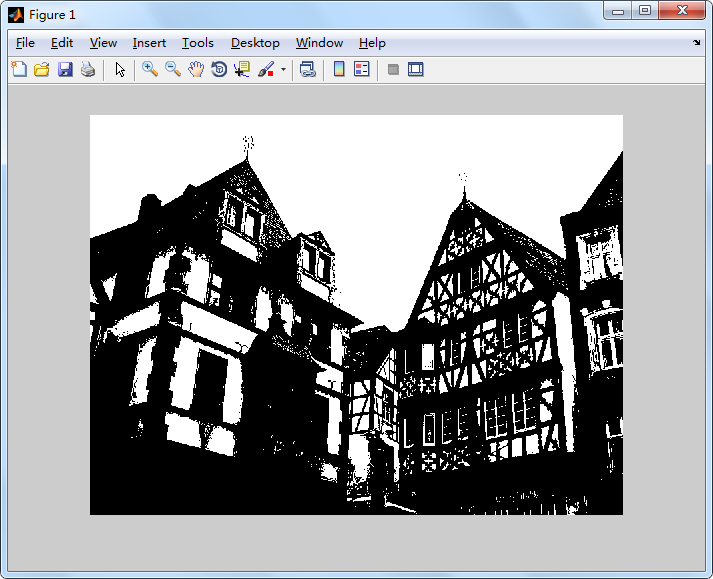更多有趣有用的图像处理算法还可以参考我的《数字图像处理原理与实践（Matlab版）》。

展开全文算法 阈值分割
• OTSU算法也称最大类间差法，有时也称之为大津算法，被认为是图像分割中阈值选取的最佳算法，计算简单，不受图像亮度和对比度的影响，因此在数字图像处理上得到了广泛的应用。它是按图像的灰度特性,将图像分成背景和...
OTSU算法也称最大类间差法，有时也称之为大津算法，被认为是图像分割中阈值选取的最佳算法，计算简单，不受图像亮度和对比度的影响，因此在数字图像处理上得到了广泛的应用。它是按图像的灰度特性,将图像分成背景和前景两部分。背景和前景之间的类间方差越大,说明构成图像的两部分的差别越大,当部分前景错分为背景或部分背景错分为前景都会导致两部分差别变小。因此,使类间方差最大的分割意味着错分概率最小。
设灰度图像灰度级是L，则灰度范围为[0,L-1]，利用OTSU算法计算图像的最佳阈值为：
t = Max[w0(t) * (u0(t) - u)^2 + w1(t) * (u1(t) - u)^2)]
其中的变量说明：当分割的阈值为t时，w0为背景比例，u0为背景均值，w1为前景比例，u1为前景均值，u为整幅图像的均值。
使以上表达式值最大的t，即为分割图像的最佳阈值。
以下是一段在OpenCV中实现的C语言程序，即一个使用OTSU算法提取图像阈值的函数，输入参数为一个图像指针，返回分割该图像的最佳阈值。
int otsuThreshold(IplImage *frame)
{
int width = frame->width;
int height = frame->height;
int pixelCount[GrayScale];
float pixelPro[GrayScale];
int i, j, pixelSum = width * height, threshold = 0;
uchar* data = (uchar*)frame->imageData;
for(i = 0; i < GrayScale; i++)
{
pixelCount[i] = 0;
pixelPro[i] = 0;
}
//统计灰度级中每个像素在整幅图像中的个数
for(i = 0; i < height; i++)
{
for(j = 0;j < width;j++)
{
pixelCount[(int)data[i * width + j]]++;
}
}

//计算每个像素在整幅图像中的比例
for(i = 0; i < GrayScale; i++)
{
pixelPro[i] = (float)pixelCount[i] / pixelSum;
}
//遍历灰度级[0,255]
float w0, w1, u0tmp, u1tmp, u0, u1, u,
deltaTmp, deltaMax = 0;
for(i = 0; i < GrayScale; i++)
{
w0 = w1 = u0tmp = u1tmp = u0 = u1 = u = deltaTmp = 0;
for(j = 0; j < GrayScale; j++)
{
if(j <= i)   //背景部分
{
w0 += pixelPro[j];
u0tmp += j * pixelPro[j];
}
else   //前景部分
{
w1 += pixelPro[j];
u1tmp += j * pixelPro[j];
}
}
u0 = u0tmp / w0;
u1 = u1tmp / w1;
u = u0tmp + u1tmp;
deltaTmp =
w0 * pow((u0 - u), 2) + w1 * pow((u1 - u), 2);
if(deltaTmp > deltaMax)
{
deltaMax = deltaTmp;
threshold = i;
}
}
return threshold;}

**********************************************************************************************
当然，我们也可以用“局部二值化”来做，具体方法参见“A License Plate-Recognition Algorithm for Intelligent Transportation System Applications.pdf”。

展开全文算法 语言 c float 图像处理
• OTSU算法也称最大类间差法，有时也称之为大津算法，由大津于1979年提出，被认为是图像分割中阈值选取的最佳算法，计算简单，不受图像亮度和对比度的影响，因此在数字图像处理上得到了广泛的应用。它是按图像的灰度...
OTSU算法

OTSU算法也称最大类间差法，有时也称之为大津算法，由大津于1979年提出，被认为是图像分割中阈值选取的最佳算法，计算简单，不受图像亮度和对比度的影响，因此在数字图像处理上得到了广泛的应用。它是按图像的灰度特性,将图像分成背景和前景两部分。因方差是灰度分布均匀性的一种度量,背景和前景之间的类间方差越大,说明构成图像的两部分的差别越大,当部分前景错分为背景或部分背景错分为前景都会导致两部分差别变小。因此,使类间方差最大的分割意味着错分概率最小。

原理：

对于图像I(x,y)，前景(即目标)和背景的分割阈值记作T，属于前景的像素点数占整幅图像的比例记为ω0，其平均灰度μ0；背景像素点数占整幅图像的比例为ω1，其平均灰度为μ1。图像的总平均灰度记为μ，类间方差记为g。

假设图像的背景较暗，并且图像的大小为M×N，图像中像素的灰度值小于阈值T的像素个数记作N0，像素灰度大于阈值T的像素个数记作N1，则有：
ω0=N0/ M×N        (1)
ω1=N1/ M×N        (2)
N0+N1=M×N        (3)
ω0+ω1=1　　　  (4)
μ=ω0*μ0+ω1*μ1 (5)
g=ω0(μ0-μ)^2+ω1(μ1-μ)^2 (6)
将式(5)代入式(6),得到等价公式:
g=ω0ω1(μ0-μ1)^2 　　       (7)

这就是类间方差。采用遍历的方法得到使类间方差g最大的阈值T,即为所求。

Ostu方法可以形象地理解为：求取直方图有两个峰值的图像中那两个峰值之间的低谷值 T 。

Otsu算法实现：

opencv函数实现：
po下opencv的源码：

 getThreshVal_Otsu_8u( const Mat& _src )
{
Size size = _src.size();
if( _src.isContinuous() )
{
size.width *= size.height;
size.height = 1;
}
const int N = 256;
int i, j, h[N] = {0};
for( i = 0; i < size.height; i++ )
{
const uchar* src = _src.data + _src.step*i;
j = 0;
#if CV_ENABLE_UNROLLED
for( ; j <= size.width - 4; j += 4 )
{
int v0 = src[j], v1 = src[j+1];
h[v0]++; h[v1]++;
v0 = src[j+2]; v1 = src[j+3];
h[v0]++; h[v1]++;
}
#endif
for( ; j < size.width; j++ )
h[src[j]]++;
}

double mu = 0, scale = 1./(size.width*size.height);
for( i = 0; i < N; i++ )
mu += i*(double)h[i];

mu *= scale;
double mu1 = 0, q1 = 0;
double max_sigma = 0, max_val = 0;

for( i = 0; i < N; i++ )
{
double p_i, q2, mu2, sigma;

p_i = h[i]*scale;
mu1 *= q1;
q1 += p_i;
q2 = 1. - q1;

if( std::min(q1,q2) < FLT_EPSILON || std::max(q1,q2) > 1. - FLT_EPSILON )
continue;

mu1 = (mu1 + i*p_i)/q1;
mu2 = (mu - q1*mu1)/q2;
sigma = q1*q2*(mu1 - mu2)*(mu1 - mu2);
if( sigma > max_sigma )
{
max_sigma = sigma;
max_val = i;
}
}

return max_val;
}


上面的应该是opencv 2以后的版本，之前的版本更好理解一些，这里也po一下，

int MyAutoFocusDll::otsuThreshold(IplImage *frame)
{
const int GrayScale = 256;
int width = frame->width;
int height = frame->height;
int pixelCount[GrayScale];
float pixelPro[GrayScale];
int i, j, pixelSum = width * height, threshold = 0;
uchar* data = (uchar*)frame->imageData;  //指向像素数据的指针
for (i = 0; i < GrayScale; i++)
{
pixelCount[i] = 0;
pixelPro[i] = 0;
}

//统计灰度级中每个像素在整幅图像中的个数
for (i = 0; i < height; i++)
{
for (j = 0; j < width; j++)
{
pixelCount[(int)data[i * width + j]]++;  //将像素值作为计数数组的下标
}
}

//计算每个像素在整幅图像中的比例
float maxPro = 0.0;
int kk = 0;
for (i = 0; i < GrayScale; i++)
{
pixelPro[i] = (float)pixelCount[i] / pixelSum;
if (pixelPro[i] > maxPro)
{
maxPro = pixelPro[i];
kk = i;
}
}

//遍历灰度级[0,255]
float w0, w1, u0tmp, u1tmp, u0, u1, u, deltaTmp, deltaMax = 0;
for (i = 0; i < GrayScale; i++)     // i作为阈值
{
w0 = w1 = u0tmp = u1tmp = u0 = u1 = u = deltaTmp = 0;
for (j = 0; j < GrayScale; j++)
{
if (j <= i)   //背景部分
{
w0 += pixelPro[j];
u0tmp += j * pixelPro[j];
}
else   //前景部分
{
w1 += pixelPro[j];
u1tmp += j * pixelPro[j];
}
}
u0 = u0tmp / w0;
u1 = u1tmp / w1;
u = u0tmp + u1tmp;
deltaTmp = w0 * pow((u0 - u), 2) + w1 * pow((u1 - u), 2);
if (deltaTmp > deltaMax)
{
deltaMax = deltaTmp;
threshold = i;
}
}

return threshold;
}

参考：

https://en.wikipedia.org/wiki/Otsu%27s_method

http://www.cnblogs.com/ranjiewen/p/6385564.html

http://blog.csdn.net/glouds/article/details/38976573
————————————————
版权声明：本文为CSDN博主「Naruto_Q」的原创文章，遵循 CC 4.0 BY-SA 版权协议，转载请附上原文出处链接及本声明。
原文链接：https://blog.csdn.net/piaoxuezhong/article/details/78302893

展开全文• 这个过程中，发现了OTSU算法，其实这也算是基本的图像处理算法，之前有过接触，但是放过了，毕竟没有实际的用过。由于现在面临的大多数问题是光照不够均匀（这一点是很难做到均匀的），大多数还有噪声，那么如何实现...
• OTSU一维算法，我自己的理解是自适应阈值分割...OTSU算法：就是计算出灰度图最佳阈值的算法 1.先对灰度图进行直方图计算并归一化处理，得到0-255之间每个像素在灰度图中出现的概率，即表示为某个像素在灰度图中出现了n
• Otsu算法（双阈值） 该算法就是利用otsu算法计算出两个阈值 公式 g=w0*(u0-u)^2+w1*(u1-u) ^2+ w2*(u2-u) ^2 g最大值时，就可以选出两个阈值 代码 求两个阈值 function [t1,t2]=DoubleOtsuThresh(img) % % Otsu 双...MATLAB 数字图像处理
•   作者：Steven Wang   ...图像处理上得到了广泛的应用。它是按图像的灰度特性,将图像分成背景和前景两部分。背景和前景之间的类间方差越大,说明构成图像的两部分的差别越大,当算法 语言 c float 图像处理
• 类间方差最大化阈值分割算法 (Otsu)可以自动计算图像二值化时的阈值，otsu的中心思想是阈值T应使目标与背景两类的类间方差最大。具体原理如下：  otsu是按图像的灰度特性，将图像分成背景和目标两部分。背景和目标...
• 作者:July 二零一一年二月二十六日。 ...参考：百度百科、维基百科、vc数字图像处理。...--------------------------------------------------...数字图像处理领域的二十四个典型算法及vc实现、第一章 一、256色转灰
• 这篇文章还是来自斯坦福课后作业hw2_3，主要是结合一个例子介绍otsu算法【亦称为大律算法，小日本】及其改进算法。  本文将先介绍老外的题目、解题思路及maltab解答，然后分析otsu算法步骤，末了给出opencv实现。 ...自适应阈值 opencv
• OTSU算法是由日本学者OTSU于1979年提出的一种对图像进行二值化的高效算法。大津法是一种图像灰度自适应的阈值分割算法，按照图像上灰度值的分布，将图像分成背景和前景两部分看待，前景就是我们要按照阈值分割出来的...
• OTSU算法也称最大类间差法，有时也称之为大津算法，被认为是图像分割中阈值选取的最佳算法，计算简单，不受图像亮度和对比度的影响，因此在数字图像处理上得到了广泛的应用。它是按图像的灰度特性,将图像分成背景和...算法
• 主要把图像处理的一部分经典基础算法梳理并实现了一遍，主要是用VS2013+OpenCV3.1.0做的。 主要目的：一是为了把基础巩固一下，以便更高效地看论文；二是为了工作基础。 因为以前在学习和研究的过程中发现自己基础...
• 写在前面 ...它被认为是图像分割中阈值选取的最佳算法，计算简单，不受图像亮度和对比度的影响，因此在数字图像处理上得到了广泛的应用。它是按图像的灰度特性，将图像分成背景和前景两部分。因方差...
• 图像处理应用中二值化操作是一个很常用的处理方式，例如零器件图片的处理、文本图片和验证码图片中字符的提取、车牌识别中的字符分割，以及视频图像中的运动目标检测中的前景分割，等等。 较为常用的图像二值化...
• OPENCV的二值化操作中，有一种“大津阈值处理”的方法，使用函数cvThreshold(image,image2,0,255,CV_THRESH_...OTSU算法也称最大类间差法，有时也称之为大津算法，由大津于1979年提出，被认为是图像分割中阈值选取的
• otsu为主函数 T为功能函数，直接在matlab运行即可得到刚萨雷斯版数字图像处理第三版第十章例10.16图所示
...